# Hilbert-Schmidt integral operator

(diff) ← Older revision | Latest revision (diff) | Newer revision → (diff)

A bounded linear integral operatoracting from the space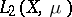into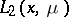and representable in the formwhere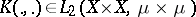is the kernel of the operator (cf. Kernel of an integral operator, ).

D. Hilbert and E. Schmidt in 1907 were the first to study operators of this kind. A Hilbert–Schmidt integral operator is a completely-continuous operator . Its adjoint is also a Hilbert–Schmidt integral operator, with kernel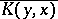. A Hilbert–Schmidt integral operator is a self-adjoint operator if and only if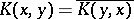for almost-all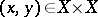(with respect to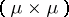). For a self-adjoint Hilbert–Schmidt integral operator and for its kernel the following expansions are valid:(1)(2)

where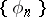is the orthonormal system of eigen functions ofcorresponding to the eigen values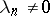. The series (1) converges with respect to the norm of, while the series (2) converges with respect to the norm of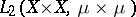, . Under the conditions of the Mercer theorem the series (2) converges absolutely and uniformly .

If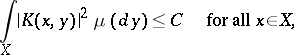then the series (1) converges absolutely and uniformly, .

Ifis a-finite measure, then the linear operator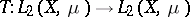is a Hilbert–Schmidt integral operator if and only if there exists a function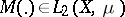such that the inequality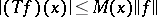is valid for almost-all(with respect to the measure) . Thus, the Hilbert–Schmidt integral operators form a two-sided ideal in the Banach algebra of all bounded linear operators acting frominto.

Hilbert–Schmidt integral operators play an important role in the theory of integral equations and in the theory of boundary value problems , , because the operators which appear in many problems of mathematical physics are either themselves Hilbert–Schmidt integral operators or else their iteration to a certain order is such an operator. A natural generalization of a Hilbert–Schmidt integral operator is a Hilbert–Schmidt operator.

How to Cite This Entry:
Hilbert-Schmidt integral operator. Encyclopedia of Mathematics. URL: http://encyclopediaofmath.org/index.php?title=Hilbert-Schmidt_integral_operator&oldid=12345
This article was adapted from an original article by B.M. Bredikhin (originator), which appeared in Encyclopedia of Mathematics - ISBN 1402006098. See original article